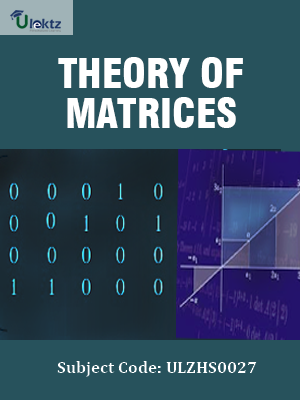The site would be on maintenance from 8th Jul 2019 2.00pm to 8:00pm. You may experience some issue during this time.uLektz apps

# Book Details# Theory of Matrices

 Course Code : ULZHS0027 Author : uLektz University : General for All University Regulation : 2013 Categories : Engineering Mathematics Format :ePUB3 (DRM Protected) Type : eBook

FREE

Description :Theory of Matrices of ULZHS0027 covers the latest syllabus prescribed by General for All University for regulation 2013. Author: uLektz, Published by uLektz Learning Solutions Private Limited.

Note : No printed book. Only ebook. Access eBook using uLektz apps for Android, iOS and Windows Desktop PC.

##### Topics
###### UNIT I THEORY OF MATRICES

1. Real matrices – Symmetric, skew – symmetric, orthogonal

2. Complex matrices: Hermitian, Skew- Hermitian and Unitary Matrices

3. Idempotent matrix, Elementary row and column transformations- Elementary matrix, Finding rank of a matrix by reducing to Echelon and normal forms

4. Finding the inverse of a non-singular square matrix using row/ column transformations (Gauss- Jordan method)

5. Consistency of system of linear equations (homogeneous and non- homogeneous) using the rank of a matrix

6. Solving m x n and n x n linear system of equations by Gauss elimination.

7. Cayley-Hamilton Theorem (without proof) – Verification. Finding inverse of a matrix and powers of a matrix by Cayley-Hamilton theorem, Linear dependence and Independence of Vectors.

8. Eigen values and eigen vectors of a matrix. Properties of eigen values and eigen vectors of real and complex matrices. Finding linearly independent eigen vectors of a matrix when the eigen values of the matrix are repeated.

9. Diagonalization of matrix -Quadratic forms up to three variables.

10. Rank – Positive definite, negative definite, semi definite, index, signature of quadratic forms

11. Reduction of a quadratic form to canonical form, Nature of quadratic forms

### Related Books